# Pipe Flow Gas Flow Rate Calculator App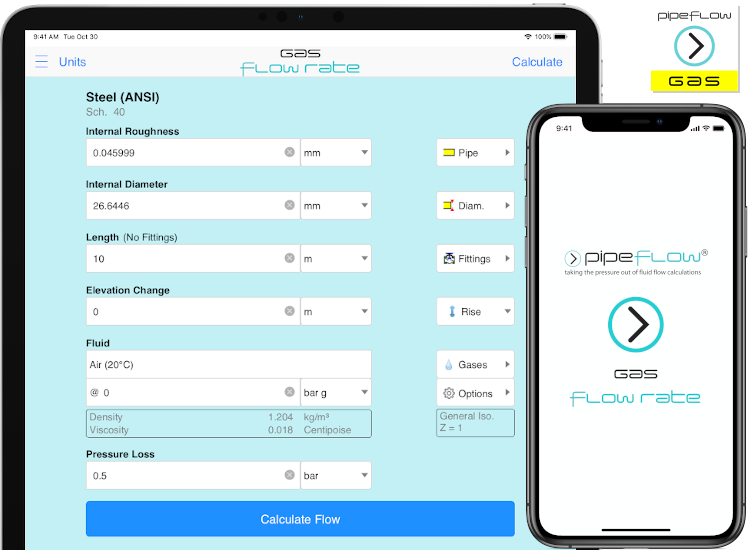## Calculate Gas Flow Rate

The Pipe Flow Gas Flow Rate App calculates the flow rate in a pipe that occurs for a given pressure difference between the start of the pipe and end of the pipe (the available pressure difference) using Compressible Isothermal Flow equations (including the General Fundamental Flow equation, Complete Isothermal Flow equation, AGA Flow equation, Weymouth Flow equation, Panhandle A Flow equation, Panhandle B Flow equation, and the IGT Flow equation). The software considers the size of the internal pipe diameter, the internal roughness of the pipe material, the length of pipe, the friction loss in the pipe for the calculated flow velocity, and the friction loss through pipe fittings & bends.

Users can select items from from the pipe database, fitting database, and fluid database, and this data can then be used in the calculation.

## Gas Flow Rate Calculation Results

Tapping "Calculate Flow Rate" calculates the gas flow rate for the specified pressure loss. The result shows the mass flow, standard flow volume, and compressed flow volume. Individual pressure losses for each item are displayed on the results screen.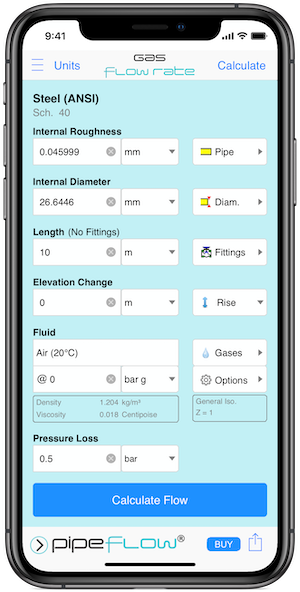Gas Flow Rate Calculation Screen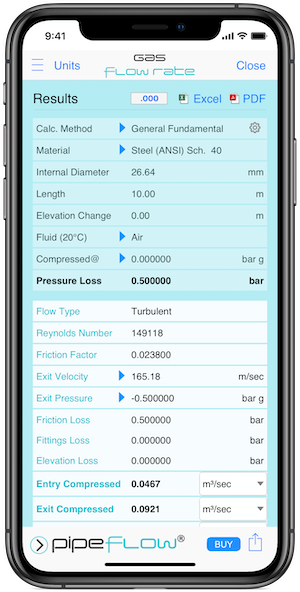Gas Flow Rate Results Screen

## Share Calculations

Calculations can easily be saved to your device or shared with other users of Pipe Flow Gas Flow Rate. The App can also generate professional PDF / Excel Reports that can be sent via email and viewed on any device.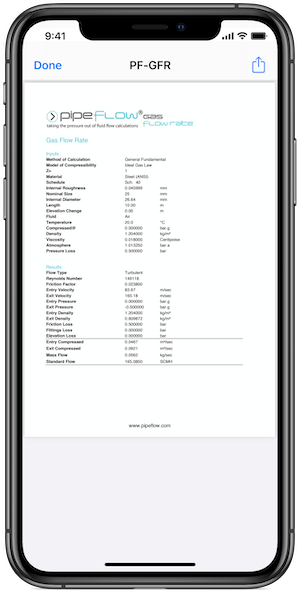Gas Flow Rate Report Screen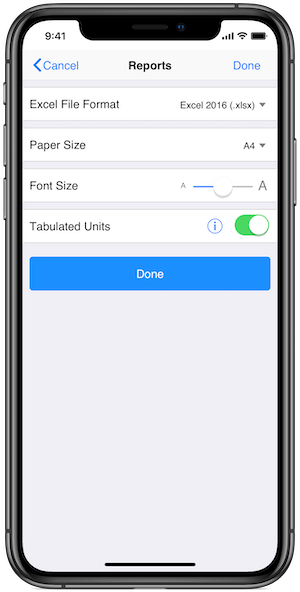Gas Flow Rate Reports Options Screen

## Choose Isothermal Gas Flow Equation & Compressibility Model

Users can select which compressible isothermal equation is used in the gas calculations:

• General Fundamental Isothermal Flow
• Complete Isothermal Flow
• AGA Isothermal Flow
• Weymouth Isothermal Flow
• Panhandle A Isothermal Flow
• Pandandle B Isothermal Flow
• IGT Isothermal Flow
By default, the calculation engine uses the Ideal Gas Law to calculate gas density at the specified pressure conditions, however if required, a custom compressibility factor or use of the CNGA compressibility factor can be used.

The software defaults to a standard atmospheric pressure of 1.01325 bar, however this can be modified by the user as required.

A range of standard volume units for gas flow rate are available within the software. The user can select their required reference condition from one of the following standard conditions:

• 0℃, 100.000 kPa.a
• 0℃, 101.325 kPa.a
• 15℃, 101.325 kPa.a
• 20℃, 101.325 kPa.a
• 25℃, 101.225 kPa.a
• 60℉, 14.696 psi.a
• 68℉, 14.696 psi.a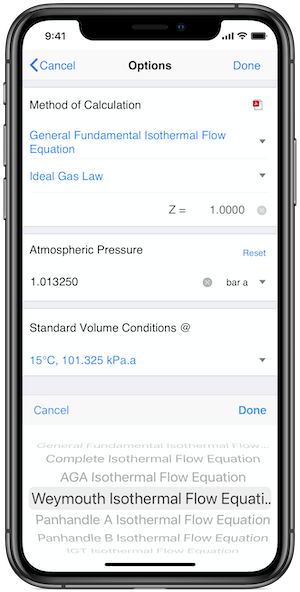Gas Options Screen

Download Pipe Flow Gas Flow Rate or scan the QR code using the camera on your device.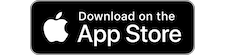Secure Online PaymentsPipe Flow Software: Piping design, Pressure drop calculator, Flow rate calculator, Pump head calculations, Pump selection software.  Copyright © Pipe Flow Software 1997-2023